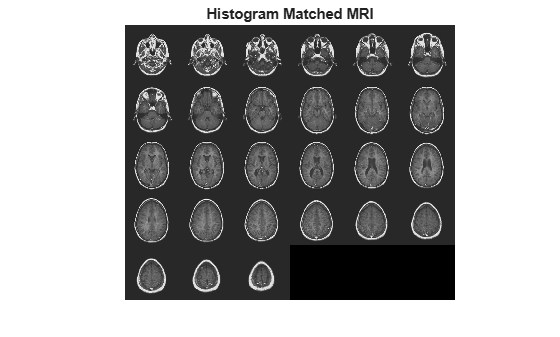imhistmatchn

Adjust histogram of N-D image to match histogram of reference image

Description

example

B = imhistmatchn(A,ref) transforms the N-D grayscale image A and returns output image B whose histogram approximately matches the histogram of the reference image ref. Both A and ref must be grayscale images, but they do not need to have the same data type, size, or number of dimensions.

B = imhistmatchn(A,ref,nbins) uses nbins equally spaced bins within the appropriate range for the given image data type. The returned image B has no more than nbins discrete levels.

If the data type of the image is:

• single or double, the histogram range is [0, 1].

• uint8, the histogram range is [0, 255].

• uint16, the histogram range is [0, 65535].

• int16, the histogram range is [-32768, 32767].

[B,hgram] = imhistmatchn(___) returns the histogram of the reference image ref used for matching in hgram. hgram is a 1-by-nbins vector, where nbins is the number of histogram bins.

Examples

collapse all

Load an N-D grayscale image into the workspace. Also load a grayscale image to provide a reference histogram.

Display the original volume as slices.

figure
montage(D,'DisplayRange',[])
title('Original 3-D Image')Reshape the reference as a stack of grayscale slices for display.

ref = reshape(mristack,[256,256,1,21]);

Display the reference volume as slices. To display correctly on the screen, the reference volume is downsized by a factor of 0.5 using imresize.

ref_downsized = imresize(ref,0.5);
figure
montage(ref_downsized,'DisplayRange',[])
title('Reference 3-D Image')Match the histogram of D to the histogram of the fullsize ref.

Dmatched = imhistmatchn(D,ref);

Display the output. Observe that the brightness levels of the output more closely match the reference image than the original image.

figure
montage(Dmatched,'DisplayRange',[])
title('Histogram Matched MRI')Input Arguments

collapse all

Input image to be transformed, specified as an N-D grayscale image.

Data Types: single | double | int16 | uint8 | uint16

Reference image whose histogram is the reference histogram, specified as a grayscale image. The reference image provides the equally spaced nbins bin reference histogram which output image B is trying to match.

Data Types: single | double | int16 | uint8 | uint16

Number of equally spaced bins in reference histogram, specified as a positive integer. nbins also represents the upper limit of the number of discrete data levels present in output image B.

Data Types: double

Output Arguments

collapse all

Output image, returned as an N-D grayscale image. The output image is derived from image A whose histogram is an approximate match to the histogram of input image ref built with nbins equally spaced bins. Image B is of the same size and data type as input image A. Input argument nbins represents the upper limit of the number of discrete levels contained in image B.

Data Types: single | double | int16 | uint8 | uint16

Histogram counts derived from reference image ref, returned as a 1-by-nbins vector.

Data Types: double